# How to recover the Borrow Bit

In a similar way as the carry bit for addition it is possible to recover the borrow bit for substraction, just based on the highest bits of three numbers that we deal with during the operation.

With this program, a subtraction operation of an 8-bit CPU can be simulated exhaustively

 #!/usr/bin/perl

 my $x,$x, $bi; my %tab = (); for ($bi = 0; $bi <= 1;$bi++) {     for ($x = 0;$x < 256; $x++) { for ($y = 0; $y < 256;$y++) {             my $zz =$x - $y -$bi;             my $b =$zz < 0 ? 1 : 0;             my $c = 1 -$b;             my $z = ($zz + 256) & 0xff;             my $xs =$x >> 7;             my $ys =$y >> 7;             my $zs =$z >> 7;             my $key = "$xs:$ys:$zs";             $tab{$key} //= $b; my$bb = $tab{$key};             if ($bb !=$b) {                 print "b=$b bb=$bb c=$c xs=$xs ys=$ys zs=$zs x=$x y=$y z=$z zz=$zz bi=$bi\n"; } } } }  for my$key (sort keys %tab) {     $key =~ m/(\d+):(\d+):(\d+)/;$xs=$1;$ys=$2;$zs=$3;$b =$tab{$key};     $c = 1 -$b;     $bb =$xs & $ys &$zs | !$xs & ($ys | $zs); print "b=$b bb=$bb c=$c xs=$xs ys=$ys zs=\$zs\n"; } 

This gives an idea, what is happening. But in real life, probably a 64bit-CPU is used, but the concepts would work with longer or shorter CPU words the same way.

So we subtract two unsigned 64-bit integers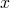and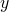and an incoming borrow bit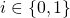to a result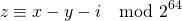with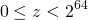using the typical „long long“ of C. We assume that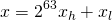where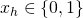and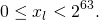In the same way we assumeand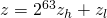with the same kind of conditions for,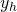,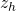or,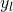,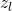, respectively.

Now we have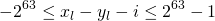and we can see thatfor some.
And we have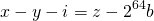where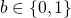is the borrow bit.
When combining we get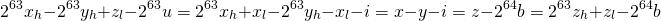When looking just at the highest visible bit and the borrow bit, this boils down to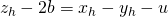This leaves us with eight cases to observe for the combination of,and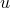:

x_hy_huz_hb
00000
00111
01011
01101
10010
10100
11000
11111

Or we can check all eight cases and find that we always haveSo the result does not depend onanymore, allowing to calculate the borrow bit by temporarily casting,and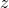to (signed long long) and using their sign.
We can express this as „use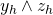if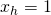and use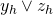if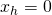„.

The incoming borrow bitdoes not change this, it just allows for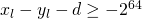, which is sufficient for making the previous calculations work.

The basic operations add, adc, sub, sbb, mul, xdiv (div is not available) have been implemented in this library for C. Feel free to use it according to the license (GPL). Addition and subtraction could be implemented in a similar way in Java, with the weirdness of declaring signed longs and using them as unsigned. For multiplication and division, native code would be needed, because Java lacks 128bit-integers. So the C-implementation is cleaner.

# Borrow and Carry Bit for Subtraction

Similar to the usage of the carry bit when adding there are mechanisms for subtracting that allow to integrate the result of subtraction of the lower bits into the subtraction of the next higher block of bits, where necessary.

There are two ways to do this, that are trivially equivalent by a simple not operation:

• borrow bit (also borrow flag)
• carry bit (also carry flag)

Often CPUs use what is the carry bit for addition and interpret it as borrow bit for subtraction.

Here are the two ways:

## Borrow Bit

It is assumed, that the CPU-word is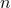bits long, so calculations are performed modulo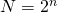. Further it is assumed, that the rangeis preferred, so all sign issues are excluded for the moment.

When subtracting two numbers,from each other like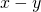and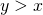, the provided result is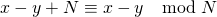and the borrow bit is set (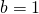), to indicate that the subtraction caused „underflow“, which had to be corrected by addedin order to get into the desired range.

In the „normal“ case where, the provided result is simply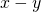and the borrow bit is not set ().

The next round of subtraction takes the borrow bit into account and calculates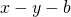, where the condition becomesand the result is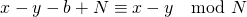or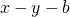respectively. This is how some of the older readers used to do it in school on paper, but of course with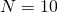.

Now the typical integer arithmetic of current CPUs uses Two’s complement, which means that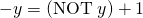. Combining this with the previous results in calculating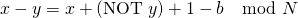At this point some CPU-designers found it more natural, to use the carry bit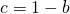instead of the borrow bit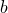.

## Carry Bit

When subtracting two numbers,from each other likeand we have, the provided result isand the carry bit is not set (), to indicate that the subtraction caused „underflow“, which had to be corrected by addedin order to get into the desired range.

In the „normal“ case where, the provided result is simplyand the carry bit is set ().

The next round of subtraction takes the borrow bit into account and calculates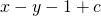, where the condition becomes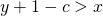and the result is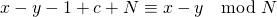orrespectively.

Now two’s complement withthis can be written asor with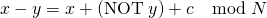These two ways are really equivalent and easily transformed into each other. Neither of them provides big advantages, apart from the fact that we have the unnecessary confusion, because it depends on the CPU design, which of the two variants is used.

## Recovery of Borrow or Carry bit

The borrow bit is calculated and used during subtractions that are done at assembly language level, but higher languages like C or Java do provide access to this information. It is relatively easy to recover the carry bit in the case of addition based on,and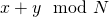.

This possible as well for the subtraction. Quite easily the comparison betweenandor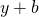could be done before the subtraction. This would work, but it is kind of inefficient, because under the hood the comparison is just a subtraction with discarding the result and keeping the flags. So the subtraction is performed twice. This might not be such a bad idea, because a compiler could recognize it or the work of subtracting twice could be neglectable compared to the logic for an „optimized“ recovery, based on some logic expression of certain bits from,and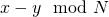.